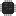# hwcalc

hwcalc, or `hc`, is a calculator for arbitrary bit-width two’s complement numbers.

It is primarily created for performing quick calculations and conversions between bases during hardware development, hence the name.

For quick conversions, `hc` will always display the result in multiple bases:

``> 49194_919 = 0b1_0011_0011_0111 = 0o1_1467 = 0x1337> 0x5391_337 = 0b101_0011_1001 = 0o2471 = 0x539``

`hc` can work with two’s complement numbers of any bit width:

``> 5 + 0b1000i4-3 (= 13) = 0b1101 = 0o15 = 0xd> -38-38 = 0b(1)01_1010 = 0o(7)32 = 0x(f)da``

Other features include:

• specific or infinite bit width,
• fixed-point numbers, specific or infinite precision,
• repeating digits for input and output,
• assignable variables,
• colored input and output,